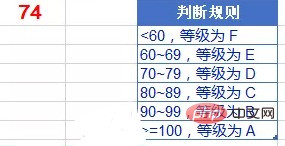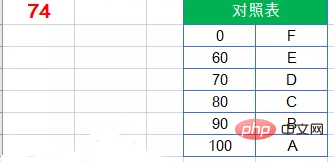﻿ Excel实例详解实现多区间判断 - 自由资讯

# Excel实例详解实现多区间判断<60，等级为“F”；

60~69，等级为“E”；

70~79，等级为“D”；

80~89，等级为“C”；

90~99，等级为“B”；

>=100，等级为“A”。## 1、IF函数

=IF(A1>=100,”A”,IF(A1>=90,”B”,IF(A1>=80,”C”,IF(A1>=70,”D”,IF(A1>=60,”E”,”F”)))))

=IF(A1<60,"F",IF(A1<70,"E",IF(A1<80,"D",IF(A1<90,"C",IF(A1<100,"B","A")))))

## 2、LOOKUP

`=LOOKUP(A1,{0,60,70,80,90,100},{"F","E","D","C","B","A"})`

LOOKUP函数以A1为查找值，返回第二参数中小于等于A1的最大数值，也就是要找所有弟弟中的大弟弟，并第三参数{“F”,”E”,”D”,”C”,”B”,”A”}中对应位置的字符串。

## 3、建立对照`=VLOOKUP(A1,D2:E7,2)`

1、是对照表中的首列使用升序排序；

2、是VLOOKUP函数省略第四参数，返回精确匹配值或近似匹配值。如果找不到精确匹配值，则返回小于待查询内容（A1）的最大值。

## 4、MATCH函数

`=MID("FEDCBA",MATCH(A1,{0,60,70,80,90,100}),1)`

MATCH函数的作用是查询某个内容在一行或一列中的位置。

MID 函数在文本字符串”FEDCBA”中截取字符串，截取的指定开始位置，就是由MATCH函数计算出的结果，截取的字符串长度为1。﻿